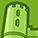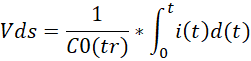Tip / Sign in to post questions, reply, level up, and achieve exciting badges. Know more

# MOSFET (Si/SiC) Forum DiscussionsLevel 3Level 3

# Co(er) vs Co(tr)

For the output capacitance Coss exist two equivalent fixed capacitance:

- Co(tr): Effective output capacitance, time related. It's a fixed capacitance that gives the same charging time as Coss while VDS is rising from 0 to 400V.

- Co(er): Effective output capacitance, energy related. It's a fixed capacitance that gives the same stored energy as Coss while VDS is rising from 0 to 400V.

The effective output capacitance is based on measurements. These values take the change of capacitance into account without the need for complex formulas.

For the dead-time calculation, e.g. for the LLC-Converter, the effective output capacitance should be used.

Why do Coss, Co(er) and Co(tr) differ so much?
Why is Co(tr) used for dead-time calculation, e.g. for the LLC-Converter, and not Co(er)?
Why is still sometimes the Coss used for the dead-time calculation?

1 SolutionModeratorModerator

# Re: Co(er) vs Co(tr)

Hi there,

Co(tr) is time related effective output capacitance, whereas Co(er) is the energy related effective output capacitance. For dead calculation, it is important to know that in how much time the output capacitance will charge, and dead time should be kept higher than that 'tr' time.

You might have also noticed that Co(tr) is greater than Co(er), so it will be worth taking Co(tr) for calculation of dead time.

If nothing is provided out of Co(er) and Co(tr), then maximum Coss can be taken for dead time calculation as a worst case condition.

Thanks.

3 RepliesModeratorModerator

# Re: Co(er) vs Co(tr)

Hi there,

Co(tr) is time related effective output capacitance, whereas Co(er) is the energy related effective output capacitance. For dead calculation, it is important to know that in how much time the output capacitance will charge, and dead time should be kept higher than that 'tr' time.

You might have also noticed that Co(tr) is greater than Co(er), so it will be worth taking Co(tr) for calculation of dead time.

If nothing is provided out of Co(er) and Co(tr), then maximum Coss can be taken for dead time calculation as a worst case condition.

Thanks.Level 3Level 3

# Re: Co(er) vs Co(tr)

Hello.

Thanks for the clarification.

So when calculating the dead time Co(tr) is the best choice. Else Co(er) should be used or when both aren't given the maximum Coss.

Unfortunately I still don't really get the full concept/idea of Co(tr).

Co(tr) is an equivalent fixed capacitance (unlike Coss, which is dynamic) to determine the charge/discharge time of the Coss independent of the topology. It thus describes the output capacitance required for the passive transition from the drain to the source voltage. tr is the time how long it takes to load the capacitance and therefor to get a Vds to increase from 0V to 400V. This can be used to describe a constant, time related effective current, which is used to load the Coss.

So it basically relies on measurement of the used power (current and voltage) and the time during the loading of Coss which can be translated into the Co(tr).

Co(tr)=I*tr/(2*U)

Is my understanding correct?

I don't quite get why Co(tr) is bigger than Co(er). Because both relay on the same Coss.
Also I don't get the full benefits of Co(tr) and Co(er).

Best regards.ModeratorModerator

# Re: Co(er) vs Co(tr)

Hi there,

Coss information is always present in datasheet, if Co(tr) is not available than it is good to go with Coss.

Co(tr) and Co(er) both are output capacitance, calculated based on the needs,  Co(er) is calculated to get an idea of what is the output capacitance at particular Vds and Eoss.

Eoss = 1/2*Co(er)*Vds*Vds. calculate in reverse to get an idea of effective output capacitance that holds relation Eoss and Vds.

C0(tr) is the output capacitance used to get an idea of what is the effective output capacitance that charges to voltage V at current i amps.

Co(tr) is the output capacitance that gives an idea of charging the output capacitance from 0V to say 400V at particular current operating current.